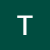# A Noob’s Guide to Random Forest

As a noob, it is very easy to get lost while understanding random forests. But fear not my fellow noobs cuz this blog will give you a starter guide to survive this tangled mess of a topic. We will also be looking at the implementation of random forest as well as decision trees on a dataset which will help you further in understanding this algorithm.

# Intern Diaries: Mathematics for ML

Today’s blog will give you a warm-up for mathematical concepts involved in machine learning. So, let’s relive those days in high-school where you absolutely “adored” mathematics and wondered how learning this stuff would be useful in life.

# Why do we need to learn maths?

• It helps you understand how certain factors,parameters and features affect the results the way they do.
• It helps you to…

# Understanding Logistic Regression

Today we will be learning about a probabilistic classification algorithm known as logistic regression and its implementation.# A Noob’s Guide to Naive Bayes

Today, we will learn about the competence of a very “naive” algorithm known as Naive Bayes. We would also be discussing its implementation (from scratch) on a dataset containing both categorical and continuous values.

# A Noob’s Guide to SVM

“Support Vector Machine” seems like handful of a name, but it is pretty simple if you understand it nicely. Today’s blog will give you a run through regarding what SVM is, how useful it is and how to implement linear SVM from scratch.

# Introduction

It is a supervised-learning algorithm used for classification and regression problems, primarily used for the former though. It can be used as a non-probabilistic linear classifier.

This means that unlike logistic regression, it doesn’t find the probability of where a data point lies. It is more straightforward than that and just gives the class in which the…

# A Noob’s Guide to K-Means

Today we will be learning together a very interesting algorithm that uses similarity among items to group them. The idea seems pretty similar to that of KNN algorithm but the algorithm is not. Let’s get started with the k-means algorithm

# What is clustering and what are its types?

• Exclusive Clustering: The clusters are non-overlapping ( example: K-means)

# A Noob’s Guide to Decision Trees

Greetings, my fellow noobs. Today I have compiled a blog for decision trees, a concept which at first glance may seem like a “tangled mess” but is much simpler than that. Here, we will grasp the idea and working of the decision tree and how it makes your life a tad bit easier.

# Introduction

It is a supervised learning algorithm used in classification and regression problems. It uses its tree-like representation to give visuals of all the decisions that can be made given the problem.

In very basic, noobish terms, a decision trees is a tree which represents all possible solutions…

# Understanding KNN Algorithm

Today, we will be learning about KNN algorithm, its applications, intuition behind it and its implementation with MNIST dataset.

# Introduction

Assume you are given 2 groups : food and vehicle. You were given the task of classifying apple in any one of the groups, then the distance between apple and the other food items would be less compared to the vehicle group. This…

# Introduction

For example,

if you are predicting whether it would rain today or not, your answer would be either “yes” or “no”. …

# Intern Diaries: Linear Algebra

In today’s blog, we will get into the nitty gritty of things and go down memory lane to revisit our “beloved” high school mathematics topic of linear algebra. We will also discuss why studying linear algebra is essential for getting started with machine learning.